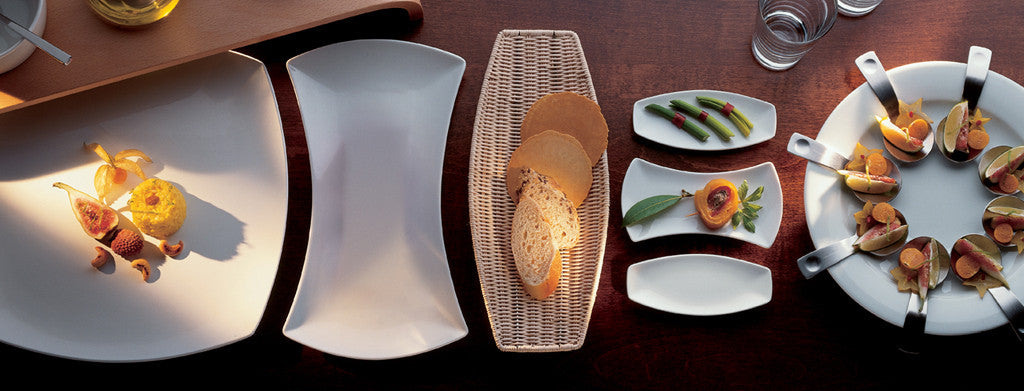Shopping Cart

### Refine

View all

#### Range# Crockery£8.95 each (ex VAT)£8.95 each (ex VAT)£4.08 each (ex VAT)£6.61 each (ex VAT)£10.22 each (ex VAT)£3.08 each (ex VAT)£2.46 each (ex VAT)£2.38 each (ex VAT)£14.46 each (ex VAT)£7.44 each (ex VAT)£2.96 each (ex VAT)£6.01 each (ex VAT)£4.49 each (ex VAT)£6.61 each (ex VAT)£4.04 each (ex VAT)£19.73 each (ex VAT)£3.29 each (ex VAT)£4.54 each (ex VAT)£7.10 each (ex VAT)£5.48 each (ex VAT)£2.68 each (ex VAT)£3.65 each (ex VAT)£4.44 each (ex VAT)£15.68 each (ex VAT)£3.75 each (ex VAT)£7.44 each (ex VAT)£6.79 each (ex VAT)£3.61 each (ex VAT)£3.85 each (ex VAT)£4.45 each (ex VAT)£3.16 each (ex VAT)£5.43 each (ex VAT)£8.72 each (ex VAT)£15.27 each (ex VAT)£12.20 each (ex VAT)£2.95 each (ex VAT)£7.59 each (ex VAT)£5.25 each (ex VAT)£15.74 each (ex VAT)£11.76 each (ex VAT)£5.78 each (ex VAT)£7.15 each (ex VAT)£1.97 each (ex VAT)£3.33 each (ex VAT)£3.23 each (ex VAT)£2.26 each (ex VAT)£1.80 each (ex VAT)£2.57 each (ex VAT)